6227.0 - Education and Work, Australia, May 2018
Previous ISSUE Released at 11:30 AM (CANBERRA TIME) 08/11/2018
 Page tools: .mffeedback,#pjs { display :none; } Enable Javascript to Print PagesPrint AllEmail NotificationRSSSearch this Product Explanatory Notes Glossary Abbreviations Quality Declaration - Summary Data quality (Technical Note) TECHNICAL NOTE RELIABILITY OF THE ESTIMATES 1 The estimates in this publication are based on information obtained from a sample survey. Any data collection may encounter factors, known as non-sampling error, which can impact on the reliability of the resulting statistics. In addition, the reliability of estimates based on sample surveys are also subject to sampling variability. That is, the estimates may differ from those that would have been produced had all persons in the population been included in the survey. NON-SAMPLING ERROR 2 Non-sampling error may occur in any collection, whether it is based on a sample or a full count such as a census. Sources of non-sampling error include non-response, errors in reporting by respondents or recording of answers by interviewers and errors in coding and processing data. Every effort is made to reduce non-sampling error by careful design and testing of questionnaires, training and supervision of interviewers, and extensive editing and quality control procedures at all stages of data processing. SAMPLING ERROR 3 Sampling error is the difference between the published estimates, derived from a sample of persons, and the value that would have been produced if the total population (as defined by the scope of the survey) had been included in the survey. One measure of the sampling error is given by the standard error (SE), which indicates the extent to which an estimate might have varied by chance because only a sample of persons was included. There are about two chances in three (67%) that a sample estimate will differ by less than one SE from the number that would have been obtained if all persons had been surveyed, and about 19 chances in 20 (95%) that the difference will be less than two SEs. 4 Another measure of the likely difference is the relative standard error (RSE), which is obtained by expressing the SE as a percentage of the estimate.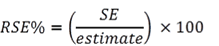5 RSEs for count estimates have been calculated using the Jackknife method of variance estimation. This involves the calculation of 30 'replicate' estimates based on 30 different subsamples of the obtained sample. The variability of estimates obtained from these subsamples is used to estimate the sample variability surrounding the count estimate. Margin of error and Confidence Intervals 8 Another useful measure is the margin of error (MOE), which shows the largest possible difference (due to sampling error) that could exist between the estimate and what would have been produced had all persons been included in the survey, at a given level of confidence. It is useful for understanding and comparing the accuracy of proportion estimates. Confidence levels can vary (e.g. typically 90%, 95% or 99%), but in this publication, all MOEs are provided for estimates at the 95% confidence level. At this level, there are 19 chances in 20 that the estimate will differ from the population value by less than the provided MOE. The 95% MOE is obtained by multiplying the SE by 1.96.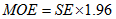9 The estimate combined with the MOE defines a range, known as a confidence interval. This range is likely to include the true population value with a given level of confidence. A confidence interval is calculated by taking the estimate plus or minus the MOE of that estimate. It is important to consider this range when using the estimates to make assertions about the population or to inform decisions. Because MOEs in this publication are provided at the 95% confidence level, a 95% confidence interval can be calculated around the estimate, as follows: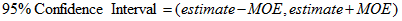USING THE MEASURES OF SAMPLING ERROR WITH THE ESTIMATES 10 This publication reports the relative standard error (RSE) for estimates of counts ('000) and the margin of error (MOE) for estimates of proportions (%). These measures are included in the datacubes available on the Downloads tab. In the first datacube (Tables 1-20: Education and Work), time series tables include both RSE of proportion and MOE of proportion, as do tables 21- 34. For years prior to 2018, MOE of proportion has been calculated using rounded figures and the result may have slightly less precision than the MOE of proportion calculated for 2018. 11 In the first datacube (Tables 1-20: Education and Work), estimates of proportions with a MOE greater than 10% are annotated to indicate they are subject to high sample variability and particular consideration should be given to the MOE when using these estimates. Depending on how the estimate is to be used, a MOE of greater than 10% may be considered too large to inform decisions. In addition, estimates with a corresponding standard 95% confidence interval that includes 0% or 100% are annotated to indicate they are usually considered unreliable for most purposes. In the remainder of the datacubes, estimates of proportions with high RSEs are annotated. The exception is 2017, where estimates of proportions are annotated based on the size of their MOE. 12 Only estimates with RSEs less than 25% are considered sufficiently reliable for most analytical purposes. All other estimates with RSEs between 25% and 50% are annotated to indicate they are subject to high sample variability relative to the size of the estimate and should be used with caution. In addition, estimates with RSEs greater than 50% are annotated to indicate they are usually considered unreliable for most purposes. 13 Caution needs to be applied when performing statistical tests for estimates on rare populations where the RSE is above 25%. In these instances, the small sample is more vulnerable to non-sampling error and the distribution of the sampling error is not symmetric around the estimate. CALCULATING MEASURES OF ERROR AND DIFFERENCE 14 Proportions or percentages formed from the ratio of two count estimates are also subject to sampling errors. The size of the error depends on the accuracy of both the numerator and the denominator. A formula to approximate the RSE of a proportion is given below. This formula is only valid when the numerator (x) is a subset of the denominator (y):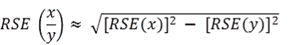15 When calculating measures of error, it may be useful to convert RSE or MOE to SE. This allows the use of standard formulas involving the SE. The SE can be obtained from RSE or MOE using the following formulas: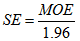16 The RSE can also be used to directly calculate a MOE with a 95% confidence level: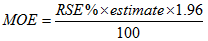Differences 17 The difference between two survey estimates (counts or percentages) can also be calculated from published estimates. Such an estimate is also subject to sampling error. The sampling error of the difference between two estimates depends on their SEs and the relationship (correlation) between them. An approximate SE of the difference between two estimates (x - y) may be calculated by the following formula: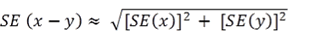18 While this formula will only be exact for differences between separate and uncorrelated characteristics or sub populations, it provides a good approximation for the differences likely to be of interest in this publication. Significance testing 19 A statistical significance test for a comparison between estimates can be performed to determine whether it is likely that there is a difference between the corresponding population characteristics. The approximate standard error of the difference between two corresponding estimates (x - y) can be calculated using the formula shown above in the Differences section. The standard error is then used to calculate the following test statistic: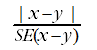20 If the value of this test statistic is greater than 1.96 then there is evidence, with a 95% level of confidence, of a statistically significant difference in the two populations with respect to that characteristic. Otherwise, it cannot be stated with confidence that there is a real difference between the populations with respect to that characteristic. Document Selection These documents will be presented in a new window.